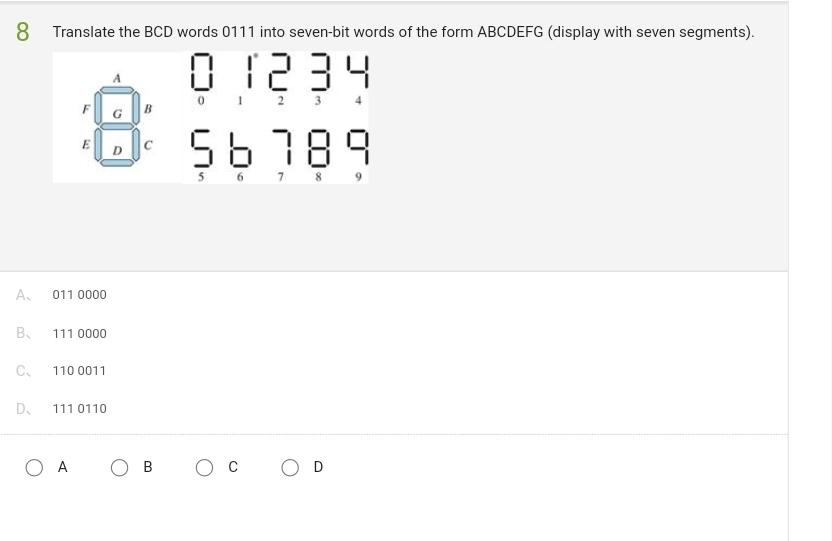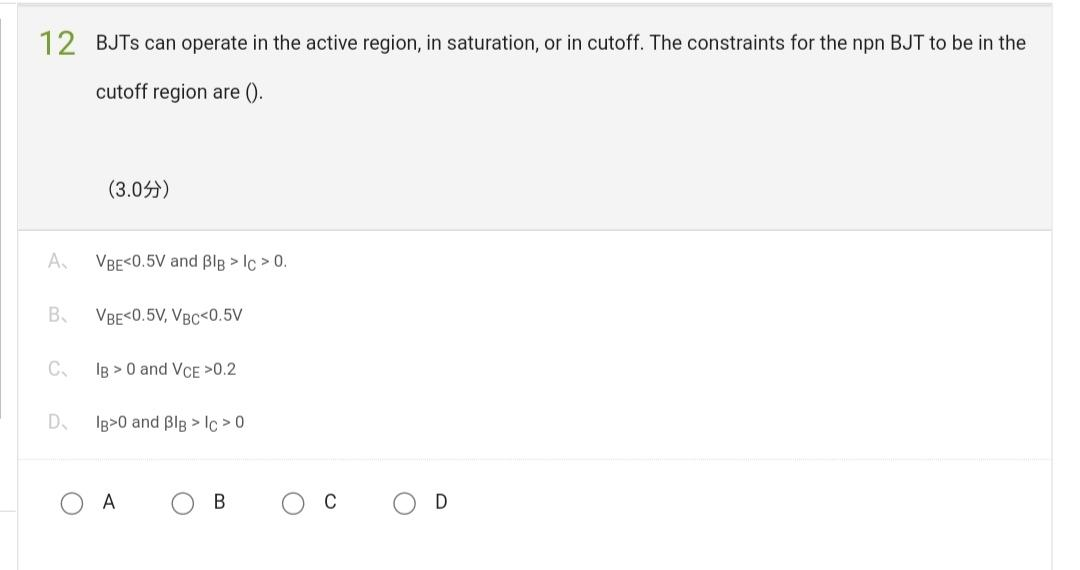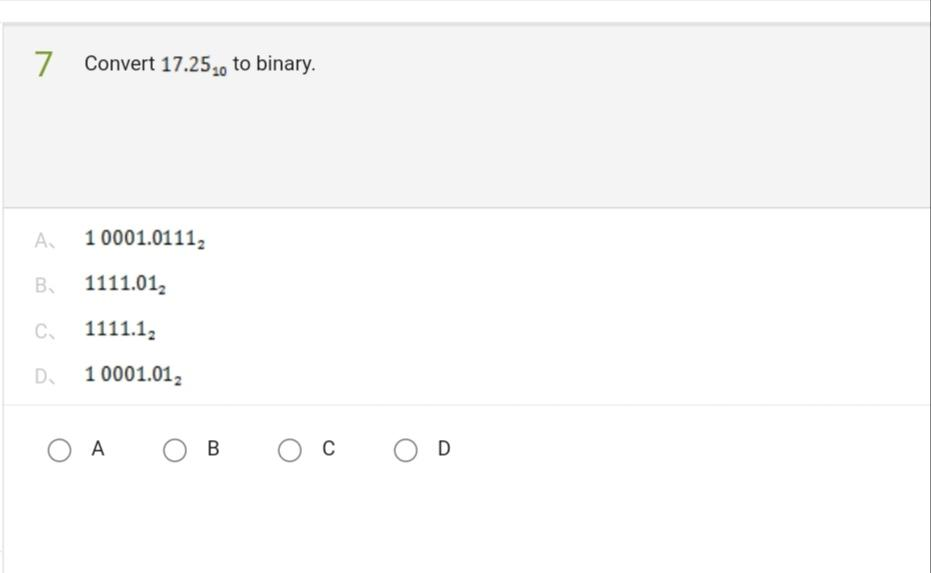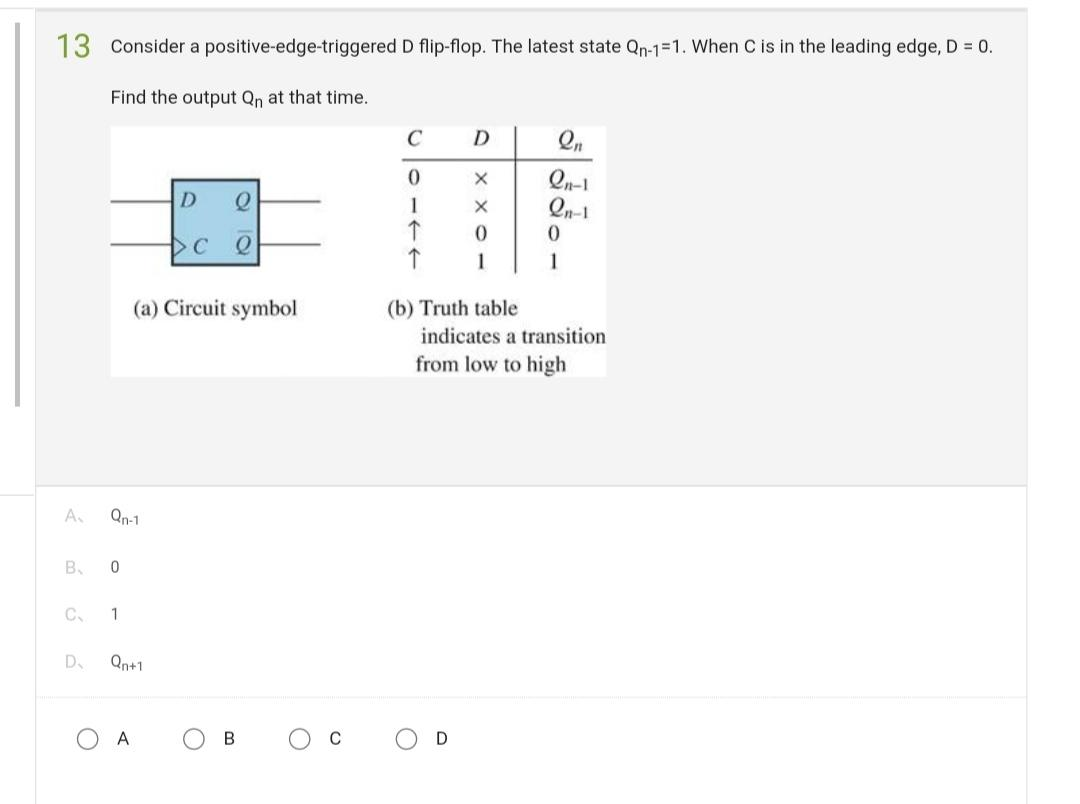# (Solved): i need this all Answer early...please solve it ..This subject is EE subject...please solve it earl ...i need this all Answer early...please solve it ..This subject is EE subject...please solve it early

Translate the BCD words 0111 into seven-bit words of the form ABCDEFG (display with seven segments). 12 BJTs can operate in the active region, in saturation, or in cutoff. The constraints for the npn BJT to be in the cutoff region are (). (3.0?) A? $$\quad V_{B E}<0.5 \mathrm{~V}$$ and $$\beta I_{B}>I_{C}>0$$. B? $$\quad V_{B E}<0.5 \mathrm{~V}, V_{B C}<0.5 \mathrm{~V}$$ C? $$I_{B}>0$$ and $$V_{C E}>0.2$$ D? $$I_{B}>0$$ and $$\beta I_{B}>I_{C}>0$$ A B C D 7 Convert $$17.25_{10}$$ to binary. A? $$10001.0111_{2}$$ B? $$\quad 1111.01_{2}$$ C? $$1111.1_{2}$$ D. $$10001.01_{2}$$ Consider a positive-edge-triggered $$D$$ flip-flop. The latest state $$Q_{n-1}=1$$. When $$C$$ is in the leading edge, $$D=0$$. Find the output $$\mathrm{Q}_{\mathrm{n}}$$ at that time. (a) Circuit symbol (b) Truth table indicates a transition from low to high

We have an Answer from Expert